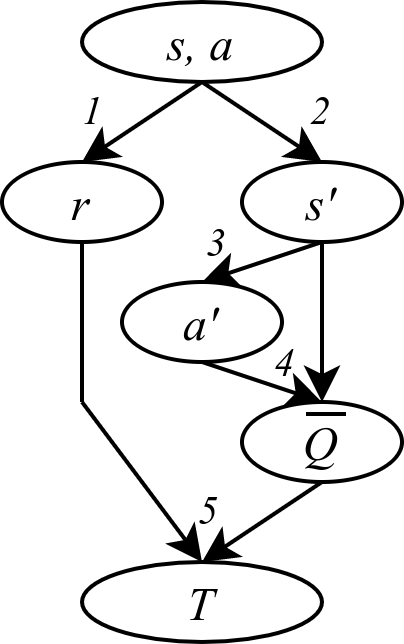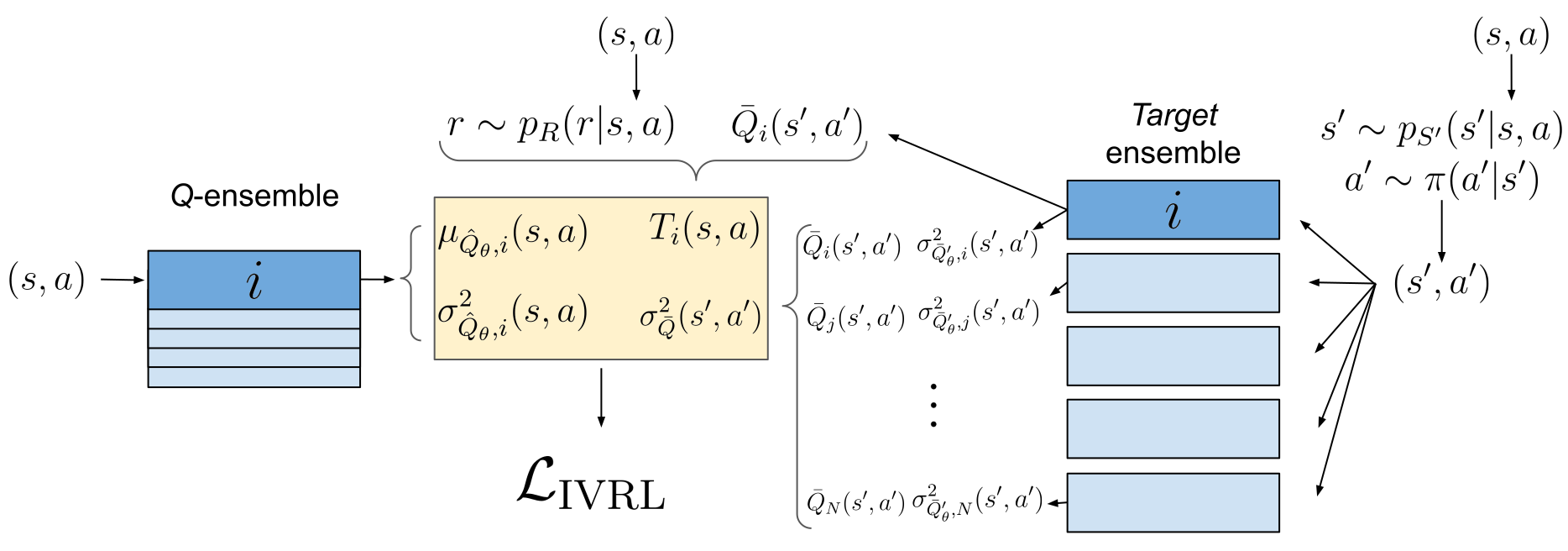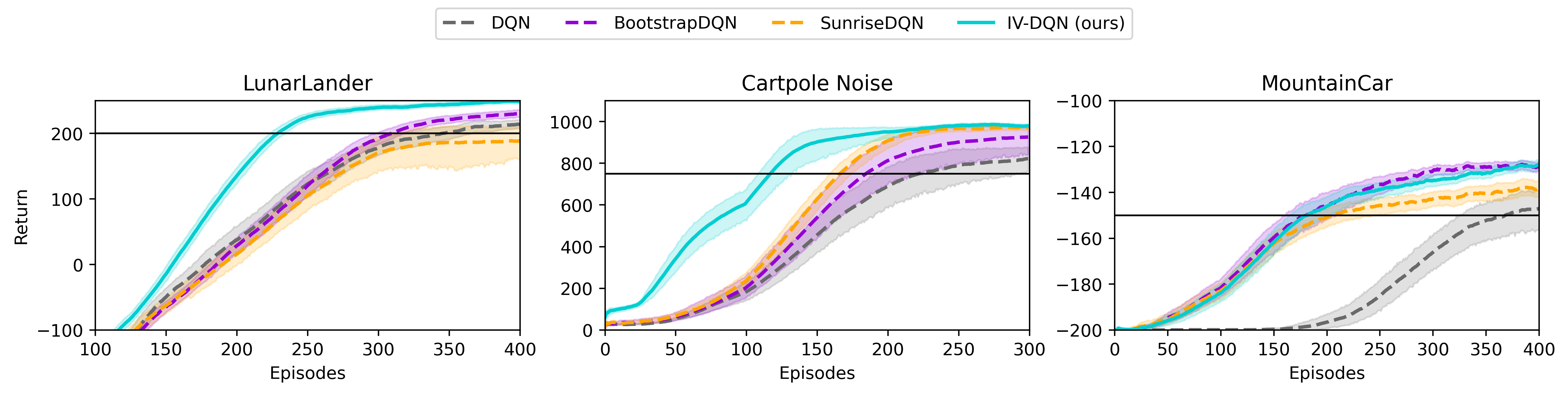Sample Efficient Reinforcement Learning Via Uncertainty Estimation

People

Vincent Mai - PhD Student

Kaustubh Mani - Past Intern, incoming PhD Student

Liam Paull - Faculty

Abstract

In model-free deep reinforcement learning (RL) algorithms, using noisy value estimates to supervise policy evaluation and optimization is detrimental to the sample efficiency. As this noise is heteroscedastic, its effects can be mitigated using uncertainty-based weights in the optimization process. Previous methods rely on sampled ensembles, which do not capture all aspects of uncertainty. We provide a systematic analysis of the sources of uncertainty in the noisy supervision that occurs in RL, and introduce inverse-variance RL, a Bayesian framework which combines probabilistic ensembles and Batch Inverse Variance weighting. We propose a method whereby two complementary uncertainty estimation methods account for both the Q-value and the environment stochasticity to better mitigate the negative impacts of noisy supervision. Our results show significant improvement in terms of sample efficiency on discrete and continuous control tasks.

Heteroschedastic noise on the targetIn most model-free deep reinforcement learning algorithms, such as DQN (Mnih et al., 2013) and SAC (Haarnoja et al., 2018), a policy $\pi$ is evaluated at state-action pair $(s,a)$. To learn how to evaluate the said policy, a neural function approximator is trained using the supervision of a target $T$. To obtain this target, the agent remembers 1) the reward $r$ it received and 2) the state $s’$ it reached after taking action $a$ at state $s$. The agent then 3) imagines what action $a’$ would be given by the policy at $s’$, and uses its current (and imperfect) policy evalution to 4) obtain the Q-value at (s’,a’). Then, the target $T$ is computed 5) by: $T(s,a) = r + \gamma \bar{Q}(s’,a’)$.

Steps 1, 2 and 3 can be stochastic, and step 4 is based on the current (and uncertain) estimation of the Q-value. These create noise on the labels of the supervision task: learning to predict the policy value. Such noisy supervision slows down the learning.

The key insight of the project is that the noise on the target is heteroschedastic, i.e. that it is not always sampled from the same distribution. If the variance of this distibution is known, it is possible to mitigate the effects of the noisy supervision by applying a weighting scheme which downweights high-variance samples, and thus to improve the sample efficiency of DRL algorithms.

The two main contributions of Inverse-Variance Reinforcement Learning (IV-RL) are thus the evaluation of the variance of the target distribution, and the use of the appropriate weighting scheme.

Evaluating the variance of the target

We evaluate the variance of the target from two ends. First, we use variance networks, trained using the negative log likelihood (NLL) loss function. $\mathcal{L}_{NLL} (x, y, \theta) = \dfrac{|f(x,\theta) - y|^2}{\sigma^2} + \log(\sigma^2)$

In supervised learning, the NLL loss leads the network to predict the variance of the labels for a given input. For example, if we have several labels for the same input (s,a), the network’s prediction will tend towards $\mu(s,a)$, the mean of the labels, and the network’s variance prediction will be the variance of these labels.

We use variance networks with NLL to estimate the variance in the target due to the stochasticity of steps 1, 2, and 3. For a better insight, assume for a moment that the value of $\bar{Q}(s’,a’)$ used in the target is always the same given (s’, a’). In that case, for a given state (s,a), having different targets T will be due to getting different values of $r$, $s’$, and $a’$. The variance output by NLL thus captures the effect on the target of the stochasticity of the reward (1), of the environment dynamics (2), and of the policy (3).

We also want to estimate the predictive uncertainty $\bar{Q}(s’,a’)$, which is bootstrapped in step 4. Due to lack of experience, this value is noisy. For example, at the beginning of the training process, the value estimator has no idea of the true value of (s’,a’). Here, we used variance ensembles [REF]: instead of a single variance network, we train 5 variance networks separately, with differences in the initial conditions and training sets. Each network predicts a mean and a variance for $\bar{Q}(s’,a’)$. The variance of $\bar{Q}(s’,a’)$ is then computed by computing the the variance of the resulting mixture of Gaussians. The intuition here is that the networks will only converge in their predictions if they have been sufficiently trained on the surroundings of (s’,a’). Otherwise, their predictions will diverge, producing a higher variance.The different steps of IV-RL. From a given $(s,a)$, the values of $r$, $s'$ and $a'$ are sampled in the environment. The Q- and target-ensembles then produce a prediction, a target, and two variance estimations which are fed to the IV-RL loss.

Weighting scheme and loss function

Once we have these two variance measures for a single target, how can we use them to mitigate the effect of the noise? In the case of heteroschedastic regression, when the variance is known, we can use weights in the loss function to reduce the impact of labels with a high noise, while trusting more the labels with a low amount of noise. In the case of linear regression, the optimal weights are the inverse of the variance. It is harder to prove they are optimal in the case of neural networks optimization, but we can follow the same idea.

In the NLL formulation for variance networks, the error is already divided by the predicted variance. We show in the paper that the simple use of variance networks in the ensemble leads to significant improvements in the sample efficiency and learning performance.

To account for the predictive uncertainty, we use a weighting scheme called Batch Inverse-Variance (BIV) (Mai et al., 2021). The inverse variance weights are normalized over the mini-batch and a hyperparameter allows to control the intensity of the weighting scheme through the effective batch size. This allows robustness, especially as the variance estimation methods we use do not always work smoothly: the variance can be strongly under or over estimated. Once again, we show that using only BIV with ensembles (without variance ensembles) improves the sample efficiency.

We combine both in a multi-objective loss function, leading to consistently better performances than when only considering one or the other uncertainty prediction.

Framework and existing work

We applied Inverse Variance RL (IV-RL) on DQN and SAC. This implementation requires a lot of design choices. In addition, ensembles can also be used for uncertainty-aware exploration strategies, which we used although they are not our contribution. We built our IV-RL framework upon existing works, in particular: BootstrapDQN (Osband et al., 2016), Randomized Prior Functions (Osband et al., 2018) and SUNRISE (Lee et al., 2020). Also note that UWAC (Wu et al., 2021) is a very interesting piece of work in the same direction than IV-RL, although looking at the slightly different problem of batch RL under the angle of out-of-distribution sample detection.

Results

IV-RL shows a significant improvement in the sample efficiency and the learning performance when applied on DQN and SAC, as shown in the figures below.IV-RL on DQN or SAC improves the learning performance and the sample efficiency compared to other ensemble-based baselines. Source: Sample Efficient Deep Reinforcement Learning via Uncertainty Estimation

More results are available in the paper and the additional contents, including ablation studies, studies of the influence of some hyperparameters, variance estimation plots, and performance of other architectures and weighting schemes.

Significance and further work

In IV-RL, the Bellman update is seen under the angle of noisy and heteroschedastic regression. This leads to the challenges of estimating the target variance and using it to reduce the effect of noise. The significant improvements shown in the results prove that this angle of analysis is a direction in which further work could help the field of DRL overcome the challenge of sample efficiency.

Implementing these insights is difficult and we had to do many compromises. Although IV-RL showcases the first, as far as we know, successful use of variance networks as an uncertainty estimation tool in DRL, the variance estimation techniques we used are tricky. They are subject to counter-intuitive learning dynamics and the scale of the predicted variances is not calibrated. To integrate them into the IV-RL algorithms, we had to take several steps to improve the robustness to over or under-estimated variance values - sometimes, having to tradeoff computational efficiency or weight discrimination power.
In addition, due to the NLL formulation already using inverse-variance weights, we were not able to merge the two estimated variances into a single objective loss function.

IV-RL proves that taking uncertainty of the target into account in the Bellman update is a highly promising direction to improve sample efficiency in DRL. We see this work as a first step and a proof of principles, and strongly encourage further work to improve this method, as well as to adapt it to other DRL algorithms and subdomains.

Volodymyr Mnih, Koray Kavukcuoglu, David Silver, Alex Graves, Ioannis Antonoglou, Daan Wierstra, and Martin Riedmiller. Playing Atari with deep reinforcement learning. NIPS, pp. 9, 2013.

Ian Osband, Charles Blundell, Alexander Pritzel, and Benjamin Van Roy. Deep exploration via bootstrapped DQN. arXiv:1602.04621, Jul 2016.

Ian Osband, John Aslanides, and Albin Cassirer. Randomized prior functions for deep reinforcement learning. In Advances in Neural Information Processing Systems, volume 31. Curran Associates, Inc., 2018

Tuomas Haarnoja, Aurick Zhou, Pieter Abbeel, and Sergey Levine. Soft actor-critic: Off-policy maximum entropy deep reinforcement learning with a stochastic actor. arXiv:1801.01290, 2018.

Kimin Lee, Michael Laskin, Aravind Srinivas, and Pieter Abbeel. SUNRISE: A simple unified framework for ensemble learning in deep reinforcement learning. arXiv:2007.04938, Jul 2020.

Yue Wu, Shuangfei Zhai, Nitish Srivastava, Joshua Susskind, Jian Zhang, Ruslan Salakhutdinov, and Hanlin Goh. Uncertainty weighted actor-critic for offline reinforcement learning. arXiv:2105.08140, May 2021.

Vincent Mai, Waleed Khamies, and Liam Paull. Batch inverse-variance weighting: Deep heteroscedastic regression. arXiv:2107.04497, Jul 2021.